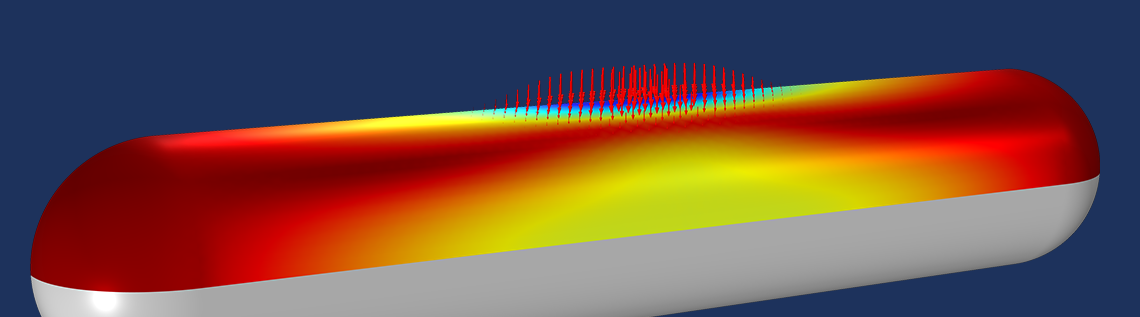# 通过符号微分加速模型收敛

2020年 10月 29日

### 利用符号微分求解简单的非线性问题

T=Q/(1+T^2/100)

r(T)=T-Q/(1+T^2/100)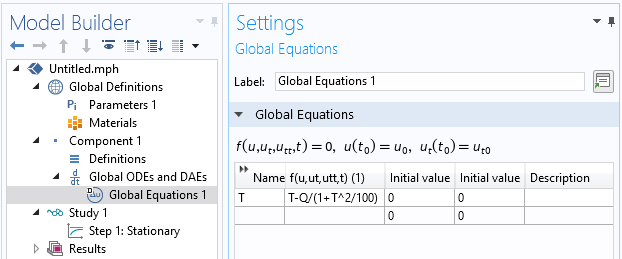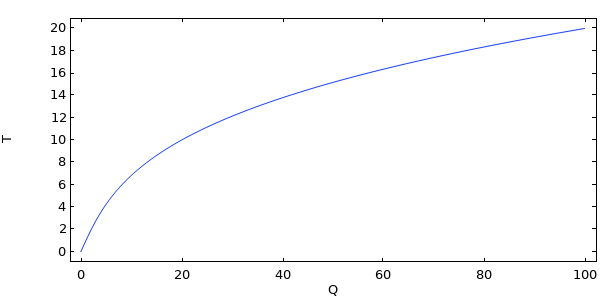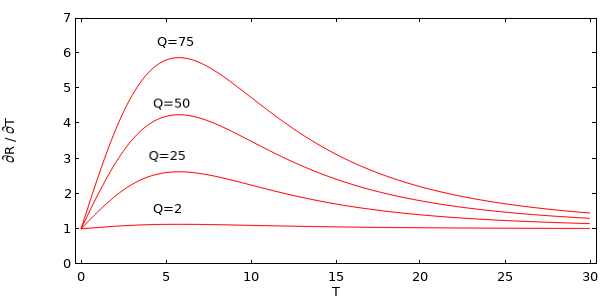\frac{\partial r(T)}
{\partial T}=1+\frac{2TQ}{100(1+T^2/100)^2}

R(T) =(T – Q/nojac(1+T^2/100))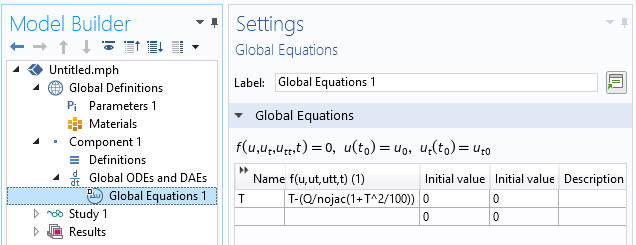\frac{\partial r(T)}{\partial T}=1

### 在 COMSOL Multiphysics® 中使用 nojac() 算子

R(T) = nojac(T – Q/(1+T^2/100))

R(T) = T – Q/(1+nojac(T)*T/100)

\frac{\partial r(T)}

{\partial T}
=1+\frac{TQ}

{100(1+T^2/100)^2}

1. 您可以使用 nojac() 算子来忽略对雅可比矩阵产生贡献的项
2. 忽略项将影响非线性求解器的收敛
3. 忽略项可减少计算模型的大小

### 减少大型非线性模型的大小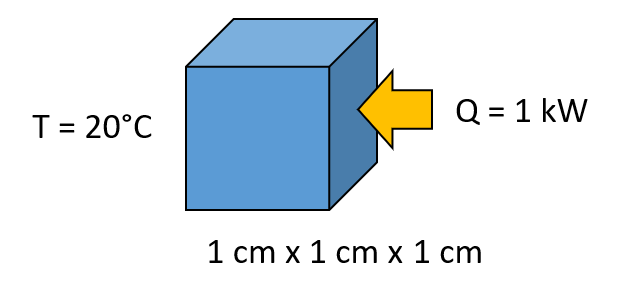(1+(T[1/K])^2/100)[W/m/K]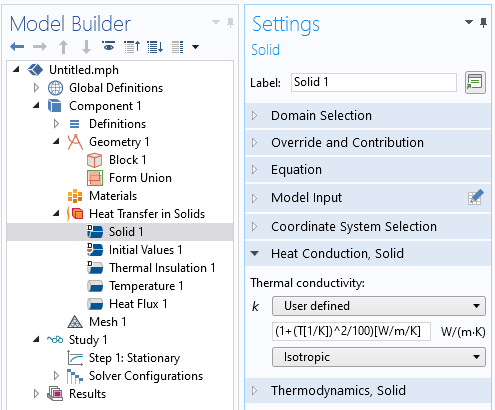找到非对称矩阵。

nojac(1+(T[1/K])^2/100)[W/m/K]

### 避免非局部耦合项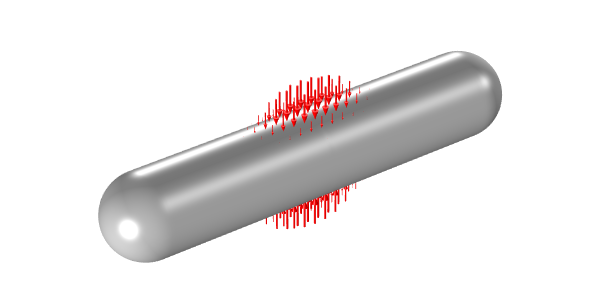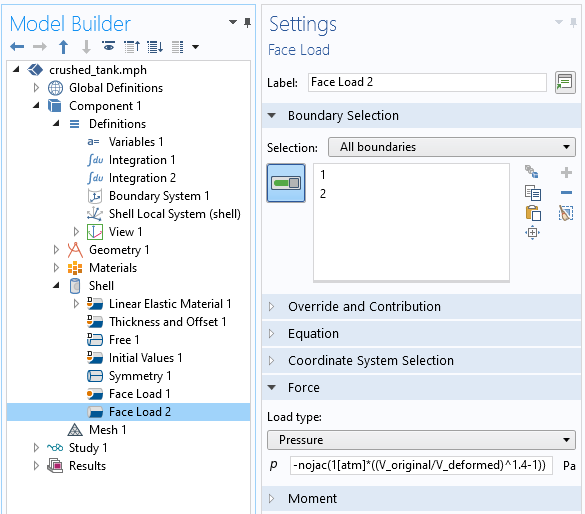### 结论

#### 评论 (4)

##### 留言##### 绍宽 毛
2022-09-14##### Haoze Wang
2022-09-16 COMSOL 员工##### 磊 张
2023-06-02##### Qihang Lin
2023-06-05 COMSOL 员工